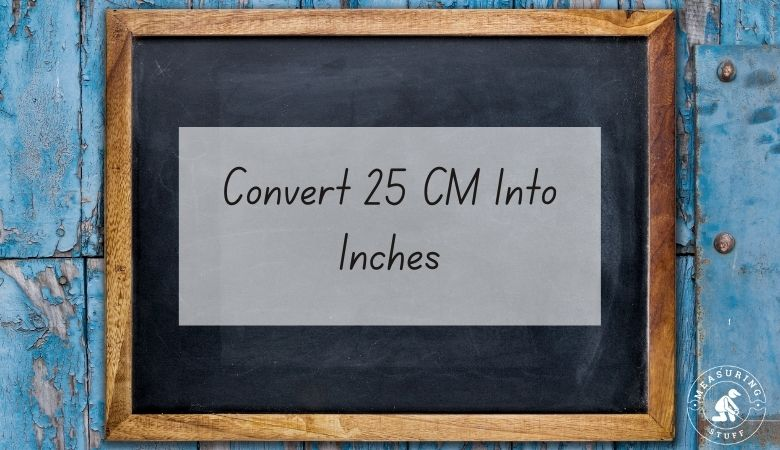# Convert 25 CM Into Inches

This article will show you how to convert 25 centimeters into inches.

There are 9.843 inches in 25 cm.

In order to find out how many inches are in a certain number of centimeters, you would need to multiply the number of centimeters by 0.394.

There are 0.394 inches in 1 centimeter, so multiplying any number of centimeters by 0.394 will give you the answer in inches.

The formula is:

# of inches = # of centimeters x 0.394

For example, if you want to know how many inches are in 25 cm, multiply 25 by 0.394.

The answer, which is 9.843, tells you that there are that many inches in 25 cm.

If you want to reverse the question and figure out how many centimeters can be made from a certain number of inches, you would divide the number of inches by 0.394.

For example, if you have 25 inches, you can divide it by 0.394 to get 63.5 centimeters.

This means that there are 63.5 cm in 25 inches.

If you don’t feel like doing the math, use our online conversion calculator below to convert different units of measurements.

## Centimeters into inches conversion calculator

Use the following calculator to easily convert cm into inches. Simply type in the desired value and select centimeters in the convert from box and inches in the convert to box. The calculator will instantly do the math for you.

Check out all our conversion calculators here.

You can also use the following table to convert centimeters into inches.

## Centimeters into inches conversion table

You can also convert 25 centimeters into other units of measurements.

• 25 cm = 250 millimeters
• 25 cm = 0.82 feet
• 25 cm = 0.25 meters

Other articles on our site using centimeters and inches include:

## Inches Measurement

According to Oxford Languages, an inch is a unit of linear measure equal to one-twelfth of a foot (2.54 cm).

Inch or inches is a length unit of measurement. It is used in the USA as a customary and Imperial unit of length.

Many people will abbreviate the word inch as in. For example, 1 inch may be written as 1 in. It can also be denoted by using the double prime symbol , for example, 1 inch can be written as 1″.

1 inch is also equal to 1⁄36 yard or 1⁄12 foot.

## Centimeters Measurement

According to Oxford Languages, a centimeter is a metric unit of length, which is a general scale of measurement used around the world. It is equal to one-hundredth of a meter.

The centimeter or cm is a decimal fraction of the meter, the International System of Units unit of length. 1 meter is equal to 39.37 inches.

## Related articles:

Check out a complete list of related articles in this category here.